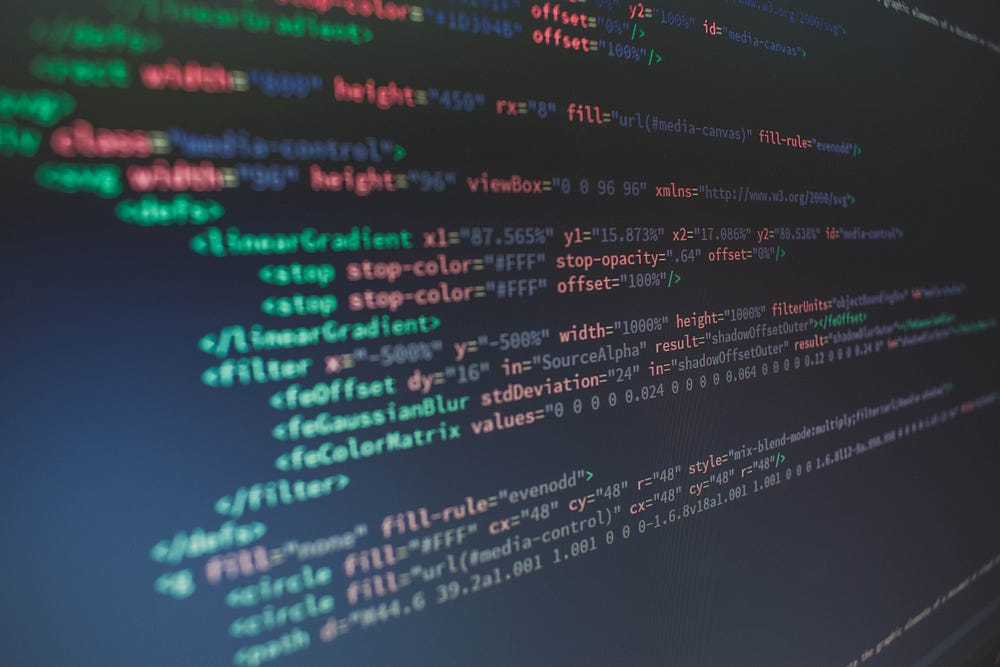# VinB AI Series Basics — 1 Python Commands

Original article was published on Artificial Intelligence on Medium# VinB AI Series Basics — 1 Python Commands

We’ll start this series called “VinB AI Series Basics” with an overview of most common Python commands used for AI programming. All articles in the Basics series would be a refresher course for ML/AI developers. If you already have experience in this domain kindly ignore and jump directly to the modeling series.

“””
This notebook will just go through the basic but the key topics in the following order:
• Numbers
• Strings
• Printing
• if, elif, else
• for Loops
• while Loops
• Range ()
• Functions
• Map
• Lambda
• Filter
• Methods

1 Numbers

print(1/2) #Division
print(2**4) #Multiplication
print(4%2) #modfunction = remainder

Result in Python as follows:-

`24.70.5162302`

2 Strings

#String example
print(“This is a python notebook”)

#Load a string to a string variable
s = ‘Lets look at Strings’
print(s)

#Find the value in the string using string position
print(s)
print(s[:4])
print(s[4:])
print(s[3:7])

Result in Python as follows:-

`This is a python notebook Lets look at Strings L Lets look at Strings s lo`

3 Printing

print(“This is python notebook”)
a=23
b=4
print(‘i have {} dogs and {} cats’.format(a,b))

Result in Python as follows:-

`This is python notebook i have 23 dogs and 4 cats`

4 if, elif, else

if 2>1:
print(‘this is perfect’)
if 1==2:
print(‘this is wrong’)
elif 3==3:
print(‘elif is good’)
else:
print(‘this is true’)

Result in Python as follows:-

`this is perfect elif is good`

5 for loops

Lst2 = [78,3,4,9,10]

for a in Lst2:
print(a)

Result in Python as follows:-

`78 3 4 9 10`

6 while loop

i=0

while i<5:
print(i)
i=i+1

Result in Python as follows:-

`0 1 2 3 4`

7 Range function

a = range(1,9)
#a gives a range object which we can use directly or in loop

for x in a:
print(x)

lst = list(range(4,8))

Result in Python as follows:-

`1 2 3 4 5 6 7 8`

8 function

def myfunc(a,b):
c= a + b
return c

def strnlp(fname,lname):
stmt = ‘My Name Is ‘+fname+’ ‘+lname
return stmt

print(myfunc(1,2))
print(strnlp(‘Vinayak’,’Bhakta’))

Result in Python as follows:-

`3 My Name Is Vinayak Bhakta`

9 map

#map — applying a function for a list of items

def sum(a):
c = a+10
return c

b = [5,10,15,20,25,30]
d = list(map(sum,b))
print(d)

def times2(x):
return x**2

v =[7,9,10,67,54,23]
out = list(map(times2,v))
print(out)

Result in Python as follows:-

`[15, 20, 25, 30, 35, 40] [49, 81, 100, 4489, 2916, 529]`

10 lambda function

#Is an anonymous function which can shorten the code instead of defining a function and calling it. In the example we show first the function and then lambda

def times2(x):
return x**2

times2(8)

times2 = lambda x:x**2

times2(8)

#Applying map to lambda function

d = list(map(lambda x:x**2,b))
print(d)

Result in Python as follows:-

`64 64 [25, 100, 225, 400, 625, 900]`

11 filter

#applying a filter where the variable is true. Only works for boolean rule

def times2(x):
return x*2 == 14

v =[7,9,10,67,54,23]

out = list(filter(times2,v))
out

``

12 method

#Are all the available functions for a particular variable.

s = ‘hello how are you’
print(s.capitalize())
print(s.isalnum())
print(s.islower())

`Hello how are you False True`